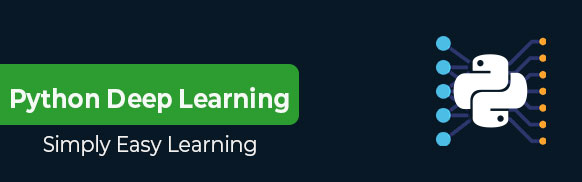# Python Deep Learning Tutorial

Python is a general-purpose high level programming language that is widely used in data science and for producing deep learning algorithms.

This brief tutorial introduces Python and its libraries like Numpy, Scipy, Pandas, Matplotlib; frameworks like Theano, TensorFlow, Keras. The tutorial explains how the different libraries and frameworks can be applied to solve complex real world problems.

# Audience

This tutorial has been prepared for professionals aspiring to learn the basics of Python and develop applications involving deep learning techniques such as convolutional neural nets, recurrent nets, back propagation, etc.

# Prerequisites

Before you proceed with this tutorial, we assume that you have prior exposure to Python, Numpy, Pandas, Scipy, Matplotib, Windows, any Linux distribution, prior basic knowledge of Linear Algebra, Calculus, Statistics and basic machine learning techniques.